Courses

WBJEE Maths Test - 9

75 Questions MCQ Test WBJEE Sample Papers, Section Wise & Full Mock Tests | WBJEE Maths Test - 9

Description
Attempt WBJEE Maths Test - 9 | 75 questions in 120 minutes | Mock test for JEE preparation | Free important questions MCQ to study WBJEE Sample Papers, Section Wise & Full Mock Tests for JEE Exam | Download free PDF with solutions
QUESTION: 1

In the expansion of (1+x)(p+q) the ratio of coefficients of xp and xq is :

Solution: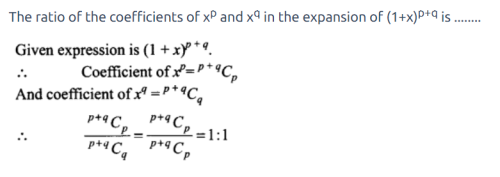QUESTION: 2

Solution:
QUESTION: 3

If 1, ω, ω2 are cube roots of unity and a + b + c = 0, then (a + bω + cω2)3 + (a + bω2 + cω)3 =

Solution:
QUESTION: 4

The locus of the centre of the circle which cuts circles x2+y2+2g₁x+2f₁y+c₁=0 and x2+y2+2g₂x+2f₂y+c₂=0 orthogonally, is

Solution:
QUESTION: 5
If the coefficient of x7 and x8 are equal in the expansion of [(2)+(x/3)]n then the value of n is :
Solution:
QUESTION: 6

If circles x2+y2+2ax+c=0 and x2+y2+2by+c=0 touch each other, then

Solution:
QUESTION: 7
If the imaginary part of [(2z+1)/(iz+1)] is -2, then the locus of z in complex plane represent.
Solution:
QUESTION: 8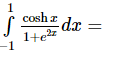Solution:
QUESTION: 9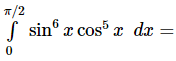Solution:
QUESTION: 10
Given 2x-y+2z=2, x-2y+z=-4, x+y+λz=4, then the value of λ such that the given system of equation has no solution, is :
Solution:
QUESTION: 11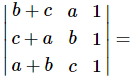Solution:
QUESTION: 12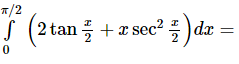Solution: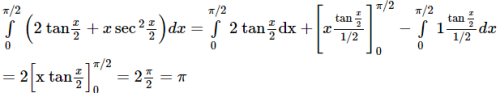QUESTION: 13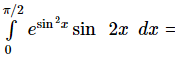Solution: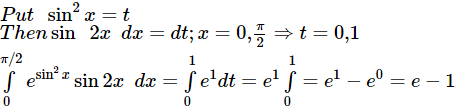QUESTION: 14

The solution of differential equation (dy/dx)=[(x(2logx+1))/(siny+ycosy)] is

Solution:
QUESTION: 15

The differential equation corresponding to the family of curves y=c(x-c)2, where c is a constant, is :

Solution:
QUESTION: 16

If f(x)=logx2log(x), then at x=e, f'(x)=

Solution:
QUESTION: 17

(d/dx)[cos⁻1((x-x⁻1)/(x+x⁻1))]=

Solution:
QUESTION: 18

The latus rectum of an ellipse is 1/3 of the major axis. It's eccentricity is

Solution:
QUESTION: 19
In an ellipse the distance between its foci is 6 and its minor axis is 8. Then its eccentricity is
Solution:
QUESTION: 20

The foci of the hyperbola 9x2 - 16y2 = 144 are

Solution:
QUESTION: 21

Equation of the tangent to the hyperbola 2x2 - 3y2 = 6 which is parallel to the line y = 3x + 4 is

Solution:

Given equation of hyperbola is 2x2 - 3y2 = 6
Dividing by 6 we get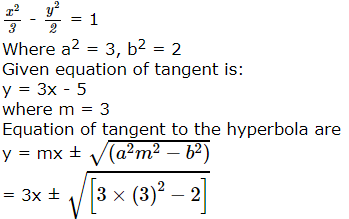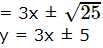Therefore equations are:
y = 3x + 5
and y = 3x - 5

QUESTION: 22

In the interval (- 3,3) the function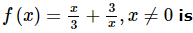Solution:
QUESTION: 23

sin⁻1[2x/(1+x)] = 2tan⁻1x for

Solution:
QUESTION: 24

The value of the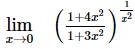is

Solution:
QUESTION: 25
A square matrix can always be expressed as a
Solution:
QUESTION: 26

Largest value of min (2 + x2, 6 - 3x) when x > 0 is :

Solution:
QUESTION: 27
The value of 0.423 is
Solution:
QUESTION: 28

The vertex of the parabola x2 + 8x + 12y + 4 = 0 is

Solution:
QUESTION: 29

The length of the latus rectum of the parabola y2 + 8x − 2y + 17 = 0 is

Solution:
QUESTION: 30
The number of ways in which 5 boys and 3 girls be seated in a row so that each girl is between two boys is
Solution: First boys are seated in 5 position in 5! Ways, now remaining 4 places can be filled by 4 girls in 4! Ways.
So number of ways = 5! 4! = 2880
QUESTION: 31
In an examination there are three objective questions and in each question there are 4 options. The number of ways in which any candidate may not give correct answers to all questions is
Solution:
QUESTION: 32

A problem in EAMCET examination is given to 3 students A, B and C whose chances of solving it are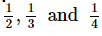respectively. The probability that the problem will be solved is

Solution:
QUESTION: 33
The number of 3 digit odd numbers, that can be formed by using the digits 1, 2, 3, 4, 5, 6 when the repetition is allowed is
Solution:
QUESTION: 34

A person puts three cards addressed to three different people in three envelopes with three different addresses without looking. What is the probability that the cards go into their respective envelopes

Solution:

Number of ways the letter can be put into envelops = 3! = 6
Probability = 1/6

QUESTION: 35
A person draws a card from a pack of playing cards, replaces it and shuffles the pack. He continues doing this until he draws a spade. The chance that he will fail in first two times is
Solution:
QUESTION: 36

In a ΔABC , the correct formulae among the following are: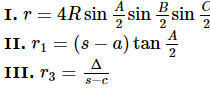Solution:
QUESTION: 37

In a ΔABC ,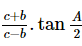is equal to

Solution: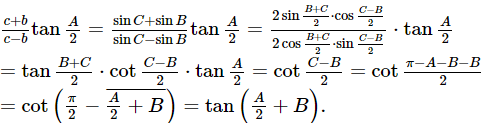QUESTION: 38

If the cube roots of unity are 1, ω, ω2 then the roots of the equation(x - 1)3 + 8 = 0, are

Solution:
QUESTION: 39

If a, b and c are real numbers such that a2 + b2 + c2 = 1, then ab + bc + ca lies in the interval

Solution:
QUESTION: 40

The absiccae of the points of the curve y = x3 in the interval [-2, 2], when the slope of the tangent can be obtained by mean value theorem for the interval [-2, 2] are

Solution:

Given that equation of curve y = x 3 = f x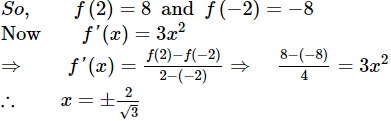QUESTION: 41

If the sum of the first n terms of a series be 5n2 + 2n, then its second term is

Solution:
QUESTION: 42

If the sum of first n terms of an A.P. be 3n2 - n and its common difference is 6, then its first term is

Solution:
QUESTION: 43
The number of terms of the A.P. 3, 7, 11, 15, ... to be taken so that the sum is 406 is
Solution:
QUESTION: 44
The sum of integers from 1 to 100 which are divisible by 2 or 5 is
Solution:
QUESTION: 45

If √3 + i = (a + i b) (c + i d) then tan⁻1 (b/a) + tan⁻1 (d/c) is equal to

Solution:
QUESTION: 46
If A, B and C are non-empty sets, then (A - B} ∪ (B - A) equals
Solution:
QUESTION: 47

The equation of bisectors between the lines 3x+4y-7=0 and 12x+5y+17=0 are

Solution:
QUESTION: 48
The line joining point (3,5) to the intersection point of lines 4x+y-1=0 and 7x-3y-35=0 is at equal distances from points (0,0) and (8,34) is the statement
Solution:
QUESTION: 49

The condition that one root of the equation ax2 + bx + c = 0 be reciprocal of other root is___

Solution:

From given hypothesis take roots α and 1/α then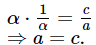QUESTION: 50
If cosθ+cos7θ+cos3θ+cos5θ=0, then θ=
Solution:
QUESTION: 51

The locus of the orthocentre of the triangle formed by the lines
(1 + p)x - py + p(1 + p) = 0,
(1 + q)x - qy + q(1 + q) = 0,
and y = 0, where p ≠ q , is

Solution:
QUESTION: 52

Let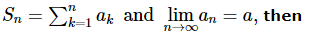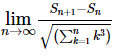Solution:
QUESTION: 53

If l is the length of the intercept made by a common tangent to the circle x2 + y2 = 16 and the ellipse x2/25 + y2/4 = 1, on the coordinate axes, then 81l2 + 3 is equal to

Solution:
QUESTION: 54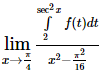equals

Solution:
QUESTION: 55

In a triangle ABC, let ∠C = π ∕ 2 . If r is the inradius and R is the circumradius of the triangle, then 2(r + R) is equal to

Solution:
QUESTION: 56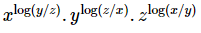equals

Solution:
QUESTION: 57

The projections of a vector on the three coordinate axis are 6, -3, 2 respectively. The direction cosines of the vector are:

Solution:
QUESTION: 58

How many real solutions does the equation x7 + 14x5 + 16x3 + 30x − 560 = 0 have?

Solution:
QUESTION: 59

f:[1, ∞) → [2, ∞) is given by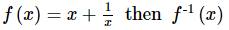equals

Solution:
QUESTION: 60

Let f (x) =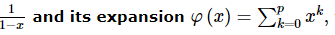then

Solution:
QUESTION: 61

Tangents are drawn from the point (- 1, 2) to y2 = 4x. The length of these tangents with intercept on line x = 2 is

Solution:
QUESTION: 62

The differential equation (x4 − 2xy2 + y4) dx − (2x2y − 4xy3 + sin y)  dy = 0 has its solution as

Solution:
QUESTION: 63

Let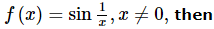f (x) can be continuous at x = 0

Solution:
QUESTION: 64

A line is drawn from A( − 2, 0) to intersect the curve y2 = 4x in P and Q in the first quadrant such that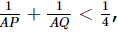then slope of the line is always

Solution:
QUESTION: 65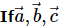are non-coplanar non-zero vectors and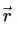is any vector in space then,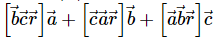is equal to

Solution:
*Multiple options can be correct
QUESTION: 66

If f(x) = x4(1-x)2, x ∈ [0,1], then which of the following is true?

Solution:
*Multiple options can be correct
QUESTION: 67

If the points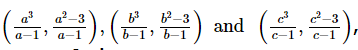where a, b, c are different from 1, lie on the line lx + my + n = 0, then:

Solution:
*Multiple options can be correct
QUESTION: 68

(m + 2) sin θ + 2(m − 1) cos θ = 2m + 1 , if:

Solution:
*Multiple options can be correct
QUESTION: 69

Let a, b, c, d real numbers. Suppose that all the roots of the equation z4 + az3 + bz2 + cz + d = 0 are complex numbers lying on the circle |z| = 1 in the complex plane. The sum of the reciprocals of the roots is necessarily

Solution:
*Multiple options can be correct
QUESTION: 70

There exist a triangle ABC satisfying the conditions,

Solution:
*Multiple options can be correct
QUESTION: 71

If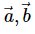are any two vectors then the vector bisecting the angle between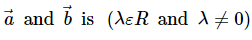Solution:
*Multiple options can be correct
QUESTION: 72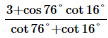is equal to

Solution:
*Multiple options can be correct
QUESTION: 73

If a, b, c are non zero real numbers such that 3 (a2 + b2 + c2 + 1)   = 2 (a + b + c + ab + bc + ca)    , then a, b, c are in

Solution:
*Multiple options can be correct
QUESTION: 74

The value of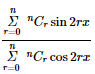is equal to

Solution:
*Multiple options can be correct
QUESTION: 75

Let f (x)  = |x − 1 | − [ x ] , then

Solution:Use Code STAYHOME200 and get INR 200 additional OFF Use Coupon Code

Track your progress, build streaks, highlight & save important lessons and more!

Similar ContentRelated tests